SSC  >  Quantitative Aptitude - Test 7

# Quantitative Aptitude - Test 7

Test Description

## 25 Questions MCQ Test SSC CGL Tier 1 Mock Test Series | Quantitative Aptitude - Test 7

Quantitative Aptitude - Test 7 for SSC 2022 is part of SSC CGL Tier 1 Mock Test Series preparation. The Quantitative Aptitude - Test 7 questions and answers have been prepared according to the SSC exam syllabus.The Quantitative Aptitude - Test 7 MCQs are made for SSC 2022 Exam. Find important definitions, questions, notes, meanings, examples, exercises, MCQs and online tests for Quantitative Aptitude - Test 7 below.
Solutions of Quantitative Aptitude - Test 7 questions in English are available as part of our SSC CGL Tier 1 Mock Test Series for SSC & Quantitative Aptitude - Test 7 solutions in Hindi for SSC CGL Tier 1 Mock Test Series course. Download more important topics, notes, lectures and mock test series for SSC Exam by signing up for free. Attempt Quantitative Aptitude - Test 7 | 25 questions in 15 minutes | Mock test for SSC preparation | Free important questions MCQ to study SSC CGL Tier 1 Mock Test Series for SSC Exam | Download free PDF with solutions
 1 Crore+ students have signed up on EduRev. Have you?
Quantitative Aptitude - Test 7 - Question 1

### A hollow sphere of internal and external radius 3 cm and 5 cm respectively is melted into a solid right circular cone of diameter 8 cm. The height of the cone is

Detailed Solution for Quantitative Aptitude - Test 7 - Question 1

We know that the formula of the volume of a hollow sphere is
4π(R3 – r3)
A hollow sphere of internal and external radius 3 cm and 5 cm respectively
So the volume of the hollow sphere = [4×π×(53 – 33)]/3 cc
Now, after melting this sphere, we will get a right circular cone, which’s diameter is 8 cm
So, radius of that cone = 8/2 cm = 4 cm
We know that the formula of the volume of a right circular cone is πr2h/3
Here, r is the radius of the cone and h is the height of the cone
From the question,
we can make the equation,
π × 42 × h/3 =  [4×π×(53 – 33)]/3
⇒ 4h = 98
⇒ h = 24.5
So, the height of the cone is 24.5 cm

Quantitative Aptitude - Test 7 - Question 2

### A and B can together do a piece of work in 28 days. If A, B and C can together finish the work in 14 days, how long will C take to do the work by himself?

Detailed Solution for Quantitative Aptitude - Test 7 - Question 2

If A and B can together do a piece of work in 28 days which means in 1 day, A and B will finish 1/28th of the work.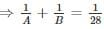If A, B and C can together finish the work in 14 days which means in 1 day, A, B and C will finish 1/14th of the work.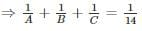Putting the first value in second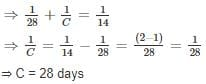Quantitative Aptitude - Test 7 - Question 3

### Two cars are moving with speeds v1, v2 towards a crossing along two roads. If their distances from the crossing be 40 metres and 50 metres at an instant of time then they do not collide if their speeds are such that

Detailed Solution for Quantitative Aptitude - Test 7 - Question 3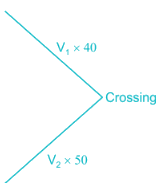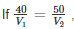then they will collide i.e. cars will reach at the same time.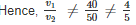Quantitative Aptitude - Test 7 - Question 4

2/5 Part of a mixture of 3 l is water and the rest is sugar syrup. Another mixture of 8 l contains 3/5 part water and the rest is sugar syrup. It both mixture are mixed together then what will be the ratio of water & sugar syrup in the new mixture?

Detailed Solution for Quantitative Aptitude - Test 7 - Question 4

Assume,
1) Mixture 1: mixture of 2/5 part water & rest sugar syrup.
2) Mixture 2: mixture of 3/5 part water & rest sugar syrup.
3) Mixture 3: mixture of mixture 1 & mixture 2.
Amount of water and sugar syrup in different mixtures are represented in tabulated form for better understanding.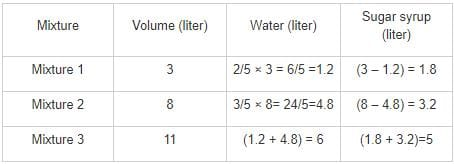∴ The ratio of water & sugar syrup in the new mixture = 6:5

Quantitative Aptitude - Test 7 - Question 5

A wire is bent in the form of an equilateral triangle encloses a region having area of 121√3 cm². If the same wire is rebent in the form of a circle, its radius will be:

Detailed Solution for Quantitative Aptitude - Test 7 - Question 5

We have area of an equilateral triangle = (√3/4) × side × side
⇒ 121√3 = (√3/4) × side × side
⇒ side × side = 121 × 4
⇒ side = 22
Perimeter = 3 × side
= 3 × 22
= 66 cm
We now have a circle with perimeter 66 cm.
⇒ 2 × (22/7) × radius = 66 cm
⇒ (44/7) × radius = 66 cm
⇒ Radius = 7 × 1.5
= 10.5 cm

Quantitative Aptitude - Test 7 - Question 6

In a class of 50 students, 20 students had an average score of 70 and remaining had an average score of 50. What is the average score of the class?

Detailed Solution for Quantitative Aptitude - Test 7 - Question 6

Total number of students = 50
20 students had an average score of 70
∴ Total score of these 20 students
= Average score × Number of students
= 70 × 20 = 1400
Remaining number of students = 50 – 20 = 30
Remainingstudents had an average score of 50
∴ Total score of these 30 students
= Average score × Number of students
= 50 × 30 = 1500
∴ Sum of scoresof these 50 students = 1400 + 1500 = 2900
∴ Average score of these 50 students
= Sum total of these 50 students/50 = 2900/50 = 58

Quantitative Aptitude - Test 7 - Question 7

In a factory 60% of the workers are above 30 years and of these 75% are males and the rest are females. If there are 1350 male workers above 30 years, the total number of workers in the factory is

Detailed Solution for Quantitative Aptitude - Test 7 - Question 7

Let the total number of workers in the factory be X. Then,
Total number of workers above 30 years of age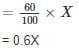Also it is given that out of these 75% are male. Thus
Total number of male workers above 30 years of age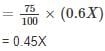It is given that total number of male workers above 30 years
= 1350
Hence,
⇒ 0.45X = 1350
⇒ X = 3000
∴ Total workers in the factory are 3000.

Quantitative Aptitude - Test 7 - Question 8

The difference between simple interest and compound interest for 2 years on the sum Rs. 2900 at a certain rate is Rs. 14.21. What is annual rate of interest?

Detailed Solution for Quantitative Aptitude - Test 7 - Question 8

We know that the difference between the simple interest (SI2) & compound interest (CI2) for 2 years [compounded annually] on a sum of Rs. P at a rate R is,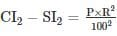Now the given information, P = Rs. 2900, CI2 – SI2 = Rs. 14.21
Putting the values we get,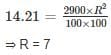The annual rate of interest is 7%

Quantitative Aptitude - Test 7 - Question 9

The third proportional to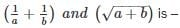Detailed Solution for Quantitative Aptitude - Test 7 - Question 9

Let, the third proportional is ‘x’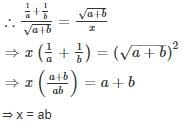Quantitative Aptitude - Test 7 - Question 10

ABC is a right angled triangle, B being the right angle. Midpoints of BC and AC are respectively B’ and A’. The ratio of the area of the quadrilateral AA’ B’B to the area of the triangle ABC is

Detailed Solution for Quantitative Aptitude - Test 7 - Question 10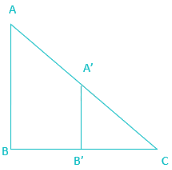Formulas:

Area of a triangle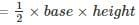Area of a trapezium =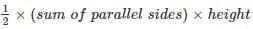Triangle ABC and A’B’C are similar by SAS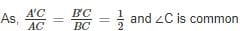By property of similar triangles,
∠B = ∠A’B’C and 2A’B’ = AB
∴ A’B’ is parallel to AB.
Thus quadrilateral AA’ B’B is a trapezium.
Height = BB’
⇒ Height = BC/2
Sum of parallel sides = (A’B’ + AB) = 3AB/2
Area of quadrilateral AA’ B’B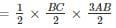⇒ Area of quadrilateral AA’ B’B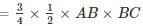Area of triangle ABC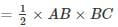Ratio area of quadrilateral AA’ B’B to area of triangle ABC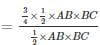⇒ Ratio of area of quadrilateral AA’ B’B to area of triangle ABC = 3 : 4

Quantitative Aptitude - Test 7 - Question 11

The cost of making an article is divided between materials, labor and overheads in the ratio 3 : 4 : 2. If the cost of material is Rs.33.60 the cost of article is

Detailed Solution for Quantitative Aptitude - Test 7 - Question 11

Let the multiplying factor be ‘x’.
Materials = 3x, Labor = 4x and Overheads = 2x
Given that, materials cost = Rs.33.60
As per sum,
⇒ 33.60 = 3x
⇒ x = 11.20
⇒ Labour = 4x = 4(11.20) = 44.80
⇒ Overheads = 2x = 2(11.20) = 22.40
∴ Cost of article = 33.60 + 44.80 + 22.40 = 100.80.

Quantitative Aptitude - Test 7 - Question 12

If a = √2 + 1, b = √2 – 1, then the value of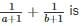Detailed Solution for Quantitative Aptitude - Test 7 - Question 12

Given,
a = √2 + 1
b = √2 – 1
Given expression is,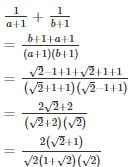= 2/2
= 1

Quantitative Aptitude - Test 7 - Question 13

If ‘a’ and ‘b’ are two odd positive integers, by which of the following is (a4 – b4) always divisible?

Detailed Solution for Quantitative Aptitude - Test 7 - Question 13

We know that, Summation and Subtraction between two odd integers gives an even integer.
Given, a and b are two odd positive integers.
∴ a + b = 2k1 ……(1)
And a – b = 2k........(2)
Where, k1 and k2are two integers.
(1) + (2) ⇒ 2a = 2(k1 + k2) ⇒ a = k1 + k2
(1) - (2) ⇒ 2b = 2(k1 - k2) ⇒ b = k1 - k2
∴ a2 + b2
= (k1 + k2)2 + (k1 - k2)2
= k12 + k22 + 2k1k2 + k12 + k22 - 2k1k2
= 2(k12 + k22) ………..(3)
Given expression is,
a4 – b4
= (a2 – b2)(a2 + b2)
= (a + b) (a - b)(a2 + b2)
Putting values from (1), (2) and (3)
= 2k× 2k2 × 2(k12 + k22)
= 8× k× k2 × (k12 + k22)
∴ a4 – b= 8× k× k2 × (k12 + k22) is always divisible by 8.

Quantitative Aptitude - Test 7 - Question 14

If (x - 2) and (x + 3) are the factors of x2 + k1x + k2 then

Detailed Solution for Quantitative Aptitude - Test 7 - Question 14

If (x - 2) and (x + 3) are the factors of the equation, then that means that
⇒ x – 2 = 0
⇒ x = 2
Similarly,
⇒ x + 3 = 0
⇒ x = -3
Putting the value of one of the x in the equation such that the value is zero.
⇒ x2 + k1x + k2
Putting the value of ‘x’ as 2, we get
⇒ (2)2 + k1(2) + k= 0
⇒ 4 +2k1+ k= 0
Putting the value of ‘x’ as -3, we get
⇒ (-3)2 + k1(-3) + k= 0
⇒ 9 -3k1+ k= 0
Subtracting both the equations:
⇒ 4 +2 k1+ k- (9 -3 k1+ k2) = 0
⇒ 4 +2 k1+ k- 9 + 3 k1- k= 0
⇒ 5 k- 5 = 0
⇒ 5 k= 5
⇒ k= 1
Putting the value of k= 1,
⇒ 4 +2 k1+ k= 0
⇒ 4 +2 (1)+ k= 0
⇒ 4 +2 + k= 0
⇒ 6 + k= 0
⇒ k= -6
Alternate method:-
(x – 2) and (x + 3) are factors of x2 + k1x + k2
∴ (x – 2)(x + 3) = x2 + k1x + k2
⇒ x2 + x – 6 = x2 + k1x + k2
Comparing, we get, k1 = 1 and k2 = - 6

Quantitative Aptitude - Test 7 - Question 15

If ab + bc + ca = 0, then the value of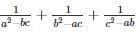Detailed Solution for Quantitative Aptitude - Test 7 - Question 15

In the given question let us add (ab + bc + ca) to all the denominators, such that the equation becomes: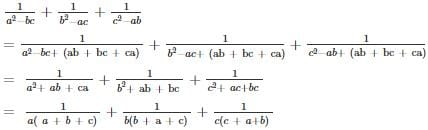Taking L.C.M of the above equation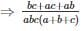⇒ 0

Quantitative Aptitude - Test 7 - Question 16

The heights of two poles are 180 m and 60 m respectively. If the angle of elevation of the top of the first pole form the foot of the second pole is 60°, what is the angle of elevation of the top of the second pole form the foot of the first?

Detailed Solution for Quantitative Aptitude - Test 7 - Question 16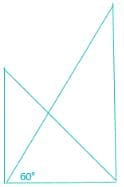Given, heights of two poles are 180 m and 60 m.
We know that the distance between the two poles acts as base and will be same so,
For the first pole,
tan 60° = height/base
⇒ √3 = 180m / b
⇒ b = 60√3
tan θ = h / 60√3
⇒ tan θ = 1/√3
⇒ θ = 30°

Quantitative Aptitude - Test 7 - Question 17

If 3 sin θ + 5 cos θ = 5, then the value of 5 sin θ – 3 cos θ wil be

Detailed Solution for Quantitative Aptitude - Test 7 - Question 17

Formula-
sin2θ + cos2 θ = 1
Given,
3 sin θ + 5 cos θ = 5 …….eq(1)
Let, 5 sin θ – 3 cos θ = x …..eq(2)
On squaring and adding both equations
(3 sin θ + 5 cos θ)2+ (5 sin θ – 3 cos θ)2 = 25 +x2
⇒ 9sinθ + 25 cosθ +30sinθ cosθ + 25 sinθ + 9cos2θ – 30sinθ cosθ = 25 +x2
⇒ 9sinθ + 9cosθ+ 25 cosθ +25 sinθ = 25 +x2
⇒ 9(sinθ +cosθ) + 25(sinθ +cosθ) =25 +x2
⇒ 9 + 25 = 25 +x2
⇒ x2= 9
⇒ x = ±3

Quantitative Aptitude - Test 7 - Question 18

A shopkeeper sold an item for Rs. 1,510 after giving a discount of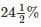and there by incurred a loss of 10%. Had he sold the item without discount, his net profit would have been

Detailed Solution for Quantitative Aptitude - Test 7 - Question 18

Given, selling price (SP) = 1510,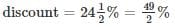Marked price – 49/2% of marked price = 1510
⇒ Marked price = 1510/0.755 = Rs. 2000
Marked price is the selling price without discount.
Given, there is a loss of 10%.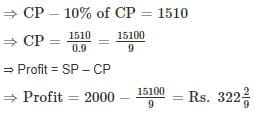Quantitative Aptitude - Test 7 - Question 19

If A(1, 2), B(4, y), C(x, 6) and D(3, 5) are the vertices of a parallelogram taken in order, then find the values of x and y.

Detailed Solution for Quantitative Aptitude - Test 7 - Question 19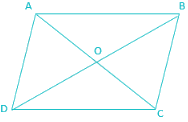Given, vertices of parallelogram are A(1, 2), B(4, y), C(x, 6) and D(3, 5)
We know that diagonals of a parallelogram bisect each other, so let the diagonals cross at point O.
Since O divides AC and BD in 1:1 ratio so,
Coordinate of O (w.r.t. AC) =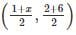Coordinate of O (w.r.t. BD)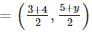Since both the coordinate s are same so equating them we get,
1 + x = 7
⇒ x = 6
And,
8 = 5 + y
⇒ y = 3

Quantitative Aptitude - Test 7 - Question 20

In a circle of radius 5 cm, AB and AC are two equal chords such that AB = AC = 6 cm. The length of BC (in cm) is

Detailed Solution for Quantitative Aptitude - Test 7 - Question 20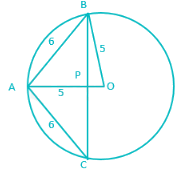Pythagoras theorem:-
Hypotenuse2 = perpendicular2 + base2
(a - b)2 = a2 + b2 - 2ab
Let O be the center of the circle.
Since Δ ABC is isosceles therefore line AO will bisect BC perpendicularly.
So, PB = CP
By Pythagoras theorem,
AB2 = AP2 + BP2
⇒ 36 = AP2 + BP2 …(1)
And,
BO2 = BP2 + OP2
⇒ 25 = BP2 + (5 – AP)2 [∵AP + OP = 5]
⇒ 25 = BP2 + 25 + AP2 – 10AP      …(2)
Substituting value of AP+ BP2 in (2)
⇒ 10AP = 36
⇒ AP = 3.6 cm
⇒ BP2 = 36 – 12.96
⇒ BP2 = 23.04
⇒ BP = 4.8 cm
⇒ BC = 9.6 cm

Quantitative Aptitude - Test 7 - Question 21

In a Δ PQR, ∠RPQ = 90°, PR = 8 cm and PQ = 6 cm, then the radius of the circumcircle of Δ PQR is

Detailed Solution for Quantitative Aptitude - Test 7 - Question 21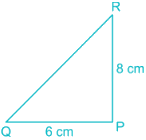Pythagoras theorem,
Hypotenuse2 = perpendicular2 + base2
Area of a triangle = abc/4R
Where, a, b and c are sides of the triangle and R is the radius of circumcircle.
Area of the triangle PQR = ½ × PQ × PR
Where PR = 8cm, PQ = 6cm
Area of triangle =12×8×6=24cm2=12×8×6=24cm2
Now, using Pythagoras theorem, a+ b2 = c2
⇒ c= (8)2 + (6)2 = 64 + 36 = 100 = (10)2
⇒ c = 10
Radius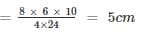Quantitative Aptitude - Test 7 - Question 22

Directions: Study the following table carefully and answer the questions.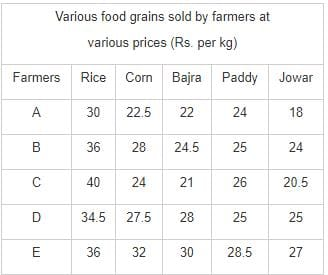Q. If farmer D and farmer E, both sell 240 kg of Bajara each, what would be the respective ratio of their earnings?

Detailed Solution for Quantitative Aptitude - Test 7 - Question 22

From the table,
Price per kg of Bajra sold by farmer D = 28
Price per kg of Bajra sold by farmer E = 30
Earning on 240 kg Bajra by D = 240 × 28 = Rs. 6720
Earning on 240 kg Bajra by E = 240 × 30 = Rs. 7200
∴ Required ratio
= 6720 : 7200 = 14 : 15

Quantitative Aptitude - Test 7 - Question 23

Directions: Study the following table carefully and answer the questions.Q.  What is the average price per kg of Bajra sold by all the farmers together?

Detailed Solution for Quantitative Aptitude - Test 7 - Question 23

Price per kg of Bajra sold by farmer A = 22
Price per kg of Bajra sold by farmer B = 24.5
Price per kg of Bajra sold by farmer C = 21
Price per kg of Bajra sold by farmer D = 28
Price per kg of Bajra sold by farmer E = 30
∴ Average price per kg of Bajra sold by all the farmers together
= (Sum of price per kg of Bajra)/number of farmer
= (22 + 24.5 + 21 + 28 + 30)/5
= 125.5/5 = 25.1

Quantitative Aptitude - Test 7 - Question 24

Directions: Study the following table carefully and answer the questions.Q. If farmer A sells 350 kg of rice, 150 kg of corn and 250 kg of jowar, how much would he earn?

Detailed Solution for Quantitative Aptitude - Test 7 - Question 24

From the table,
Price per kg of rice sold by farmer A = 30
Price per kg of corn sold by farmer A = 22.5
Price per kg of jowar sold by farmer A = 18
∴ Earning on 350 kg of rice by farmer A = 350 × 30 = Rs. 10500
Earning on 150 kg of corn by farmer A = 150 × 22.5 = Rs. 3375
Earning on 250 kg of jowar by farmer A = 250 × 18 = Rs. 4500
∴ Total earn = (10500 + 3375 + 4500) = Rs. 18375

Quantitative Aptitude - Test 7 - Question 25

Directions: Study the following table carefully and answer the questions.Q. Earnings on 150 kg of paddy sold by farmer B are approximately what percent of the earnings on the same amount of rice sold by the same farmer?

Detailed Solution for Quantitative Aptitude - Test 7 - Question 25

Given,
Price per kg of paddy sold by farmer B = 25
Price per kg of rice sold by farmer B = 36
∴ Earning on 150 kg of paddy by farmer B = 150 × 25 = Rs. 3750
Earning on 150 kg of rice by farmer B = 150 × 36 = Rs. 5400
∴ Required per cent
= (3750/5400) × 100
= 3750/54 = 69.44%

## SSC CGL Tier 1 Mock Test Series

42 docs|103 tests
 Use Code STAYHOME200 and get INR 200 additional OFF Use Coupon Code
Information about Quantitative Aptitude - Test 7 Page
In this test you can find the Exam questions for Quantitative Aptitude - Test 7 solved & explained in the simplest way possible. Besides giving Questions and answers for Quantitative Aptitude - Test 7, EduRev gives you an ample number of Online tests for practice

## SSC CGL Tier 1 Mock Test Series

42 docs|103 tests